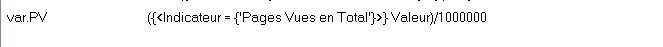# Qlik Sense App Development

Announcements
Qlik Analytics Tour 2020 Online. Begins August 10th. Register Today
cancel
Showing results for
Did you mean:
HighlightedCreator

## What is the syntax of a variable, to use it in an expression ?

Hi the community !

I would like to shorten this expression to use it in set analysis :

`sum(({<Indicateur = {'Pages Vues en Total'}>} Valeur)/1000000)`

i will need to use 'Pages Vues en Total' (=pages views)   in Sum or Avg.

i tried to create a VARIABLE   " Var.PV" =

`({<Indicateur = {'Pages Vues en Total'}>} Valeur)/1000000`

I do not manage to use ot in the expression as

`sum(Var.PV)`

Then, i will have to use this VARIABLE in that kind of expression, to compare it VS previous month :

```(
sum( {<Mois = {\$(=num(Max (Mois),'00'))}>} [VARIABLE])-sum( {<Année = {\$(=num(  if(max(Mois)=1, Max (Année)-1,Max (Année))  ,'00'))},  Mois = {\$(=num( if(max(Mois)=1, 12, max(Mois)-1) ,'00'))}>}  [VARIABLE])
)
/sum( {<Année =  {\$(=num(  if(max(Mois)=1, Max (Année)-1,Max (Année))  ,'00'))},   Mois = {\$(=num(if(Max (Mois)=1,12,max(Mois)-1),'00'))}>} [VARIABLE])```

Any idea of the syntax ?

Tags (4)
1 Solution

Accepted Solutions
HighlightedMVP & Luminary

## Re: What is the syntax of a variable, to use it in an expression ?

You need to remove the two brackets from within the variable, or from where you are using it.

When the variable is copied and pasted (effectively) into your code you get:

sum(({<Indicateur={'Pages Vues en Total'}>}Valuer)/1000000)

Because of the double bracket at the start, the Set Analysis code is not part of the sum, rather in a bracketed set of code by itself, and set analysis doesn't work like that.

Hope that makes sense?

3 Replies
HighlightedMVP & Luminary

## Re: What is the syntax of a variable, to use it in an expression ?

The syntax to use a variable is \$(), so:

sum(\$(Var.PV))

When you use a variable in this way the content of the variable is effectively copied and pasted into the location where the \$() appears.

Note that behaviour is different if your variable content begins with an equals symbol, as in this case the variable code is evaluated outside of the object where it is used and the result is returned.

\$(=some(expression)) effectively calculates the expression outside of the context where the variable is used also.

Hope that makes sense?

Instinctively, I have never put a period in any of my variable names, but I see no reason why it shouldn't work. If it doesn't maybe try just VarPV.

Steve
HighlightedCreator

## Re: What is the syntax of a variable, to use it in an expression ?

Thank you Steve for your time.

But it does not work.

These are the variable and the expression :`sum(\$(var.PV))`
HighlightedMVP & Luminary

## Re: What is the syntax of a variable, to use it in an expression ?

You need to remove the two brackets from within the variable, or from where you are using it.

When the variable is copied and pasted (effectively) into your code you get:

sum(({<Indicateur={'Pages Vues en Total'}>}Valuer)/1000000)

Because of the double bracket at the start, the Set Analysis code is not part of the sum, rather in a bracketed set of code by itself, and set analysis doesn't work like that.

Hope that makes sense?